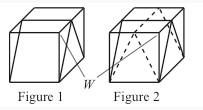###### back to index | new

A block of wood has the shape of a right circular cylinder with radius $6$ and height $8$, and its entire surface has been painted blue. Points $A$ and $B$ are chosen on the edge of one of the circular faces of the cylinder so that $\overset\frown{AB}$ on that face measures $120^\text{o}$. The block is then sliced in half along the plane that passes through point $A$, point $B$, and the center of the cylinder, revealing a flat, unpainted face on each half. The area of one of these unpainted faces is $a\cdot\pi + b\sqrt{c}$, where $a$, $b$, and $c$ are integers and $c$ is not divisible by the square of any prime. Find $a+b+c$.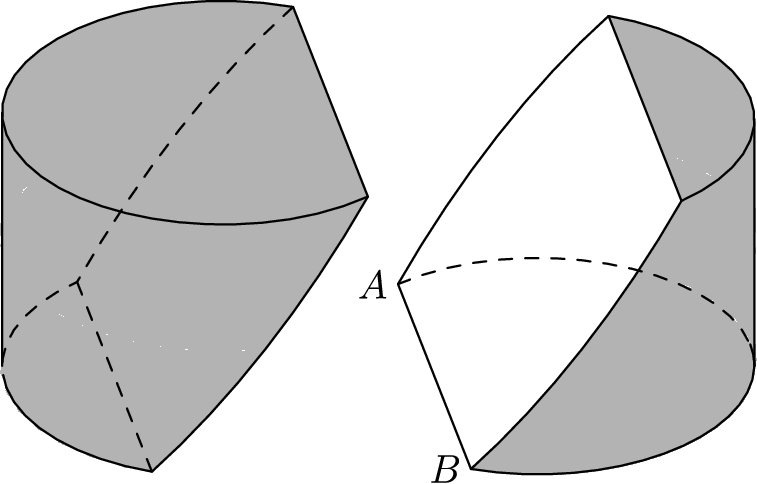A cylindrical barrel with radius $4$ feet and height $10$ feet is full of water. A solid cube with side length $8$ feet is set into the barrel so that the diagonal of the cube is vertical. The volume of water thus displaced is $v$ cubic feet. Find $v^2$.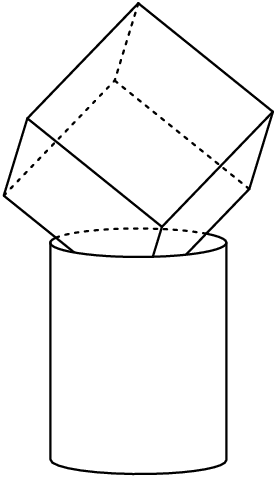A rectangular box has width $12$ inches, length $16$ inches, and height $\frac{m}{n}$ inches, where $m$ and $n$ are relatively prime positive integers. Three faces of the box meet at a corner of the box. The center points of those three faces are the vertices of a triangle with an area of $30$ square inches. Find $m+n$.

Cube $ABCDEFGH,$ labeled as shown below, has edge length $1$ and is cut by a plane passing through vertex $D$ and the midpoints $M$ and $N$ of $\overline{AB}$ and $\overline{CG}$ respectively. The plane divides the cube into two solids. The volume of the larger of the two solids can be written in the form $\tfrac{p}{q},$ where $p$ and $q$ are relatively prime positive integers. Find $p+q.$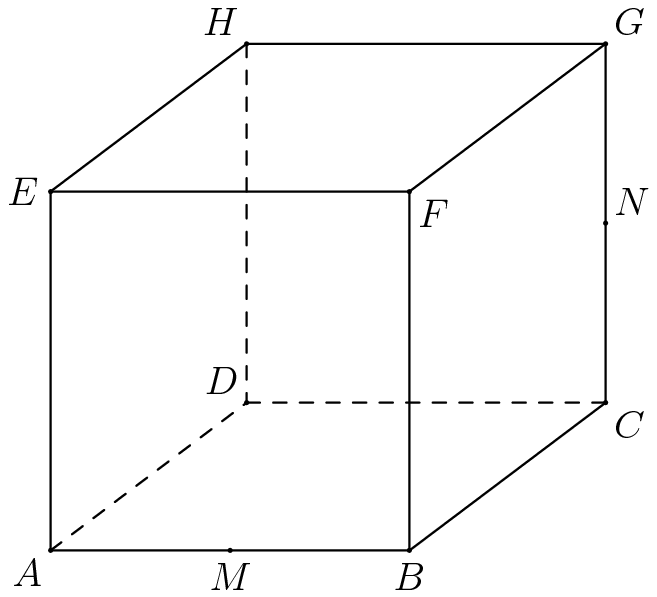In the accompanying figure, the outer square $S$ has side length $40$. A second square $S'$ of side length $15$ is constructed inside $S$ with the same center as $S$ and with sides parallel to those of $S$. From each midpoint of a side of $S$, segments are drawn to the two closest vertices of $S'$. The result is a four-pointed starlike figure inscribed in $S$. The star figure is cut out and then folded to form a pyramid with base $S'$. Find the volume of this pyramid.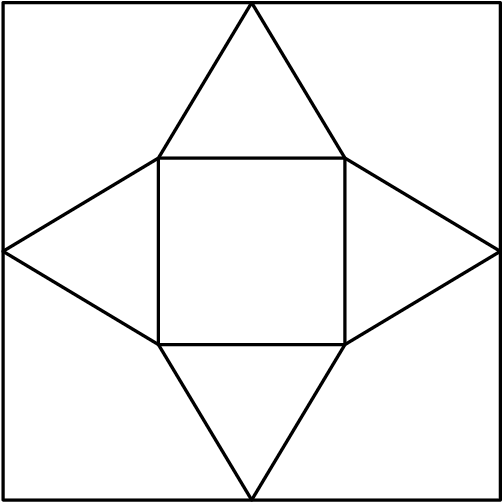A cube with side length 10 is suspended above a plane. The vertex closest to the plane is labeled $A$. The three vertices adjacent to vertex $A$ are at heights 10, 11, and 12 above the plane. The distance from vertex $A$ to the plane can be expressed as $\frac{r-\sqrt{s}}{t}$, where $r$, $s$, and $t$ are positive integers, and $r+s+t<{1000}$. Find $r+s+t$.

Let $\mathcal{R}$ be the region consisting of the set of points in the coordinate plane that satisfy both $|8 - x| + y \le 10$ and $3y - x \ge 15$. When $\mathcal{R}$ is revolved around the line whose equation is $3y - x = 15$, the volume of the resulting solid is $\frac {m\pi}{n\sqrt {p}}$, where $m$, $n$, and $p$ are positive integers, $m$ and $n$ are relatively prime, and $p$ is not divisible by the square of any prime. Find $m + n + p$.

A regular hexagon with sides of length 6 has an isosceles triangle attached to each side. Each of these triangles has two sides of length 8. The isosceles triangles are folded to make a pyramid with the hexagon as the base of the pyramid. What is the volume of the pyramid?

A $4\times 4\times h$ rectangular box contains a sphere of radius $2$ and eight smaller spheres of radius $1$. The smaller spheres are each tangent to three sides of the box, and the larger sphere is tangent to each of the smaller spheres. What is $h$?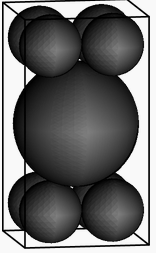A sphere is inscribed in a truncated right circular cone as shown. The volume of the truncated cone is twice that of the sphere. What is the ratio of the radius of the bottom base of the truncated cone to the radius of the top base of the truncated cone?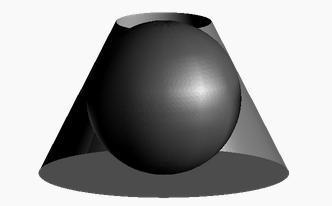The shape below can be folded along the dashed lines and taped together along the edges to form a three-dimensional polyhedron. All lengths in the diagram are given in inches. What is the volume of the resulting polyhedron? Express your answer in simplest radical form.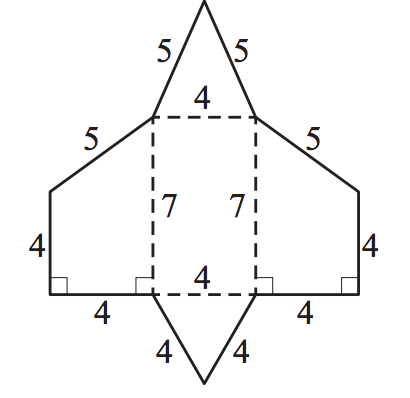Distinct planes $p_1,p_2,....,p_k$ intersect the interior of a cube $Q$. Let $S$ be the union of the faces of $Q$ and let $P =\bigcup_{j=1}^{k}p_{j}$. The intersection of $P$ and $S$ consists of the union of all segments joining the midpoints of every pair of edges belonging to the same face of $Q$. What is the difference between the maximum and minimum possible values of $k$?

Jesse cuts a circular disk of radius 12, along 2 radii to form 2 sectors, one with a central angle of 120. He makes two circular cones using each sector to form the lateral surface of each cone. What is the ratio of the volume of the smaller cone to the larger cone?

The circular base of a hemisphere of radius $2$ rests on the base of a square pyramid of height $6$. The hemisphere is tangent to the other four faces of the pyramid. What is the edge-length of the base of the pyramid?

A pyramid has a square base with side of length 1 and has lateral faces that are equilateral triangles. A cube is placed within the pyramid so that one face is on the base of the pyramid and its opposite face has all its edges on the lateral faces of the pyramid. What is the volume of this cube?

A regular octahedron has side length $1$. A plane parallel to two of its opposite faces cuts the octahedron into the two congruent solids. The polygon formed by the intersection of the plane and the octahedron has area $\frac {a\sqrt {b}}{c}$, where $a$, $b$, and $c$ are positive integers, $a$ and $c$ are relatively prime, and $b$ is not divisible by the square of any prime. What is $a + b + c$?

A convex polyhedron $Q$ has vertices $V_1,V_2,\ldots,V_n$, and $100$ edges. The polyhedron is cut by planes $P_1,P_2,\ldots,P_n$ in such a way that plane $P_k$ cuts only those edges that meet at vertex $V_k$. In addition, no two planes intersect inside or on $Q$. The cuts produce $n$ pyramids and a new polyhedron $R$. How many edges does $R$ have?

What is the volume of a cube whose surface area is twice that of a cube with volume 1?

Triangle $ABC$, with sides of length $5$, $6$, and $7$, has one vertex on the positive $x$-axis, one on the positive $y$-axis, and one on the positive $z$-axis. Let $O$ be the origin. What is the volume of tetrahedron $OABC$?

A cone-shaped mountain has its base on the ocean floor and has a height of 8000 feet. The top $\frac{1}{8}$ of the volume of the mountain is above water. What is the depth of the ocean at the base of the mountain in feet?

A pyramid has a square base $ABCD$ and vertex $E$. The area of square $ABCD$ is $196$, and the areas of $\triangle ABE$ and $\triangle CDE$ are $105$ and $91$, respectively. What is the volume of the pyramid?

An aquarium has a rectangular base that measures 100 cm by 40 cm and has a height of 50 cm. It is filled with water to a height of 40 cm. A brick with a rectangular base that measures 40 cm by 20 cm and a height of 10 cm is placed in the aquarium. By how many centimeters does the water rise?

Corners are sliced off a unit cube so that the six faces each become regular octagons. What is the total volume of the removed tetrahedra?

Rhombus $ABCD$, with side length $6$, is rolled to form a cylinder of volume $6$ by taping $\overline{AB}$ to $\overline{DC}$. What is $\sin(\angle ABC)$?

A unit cube is cut twice to form three triangular prisms, two of which are congruent, as shown in Figure 1. The cube is then cut in the same manner along the dashed lines shown in Figure 2. This creates nine pieces. What is the volume of the piece that contains vertex $W$?